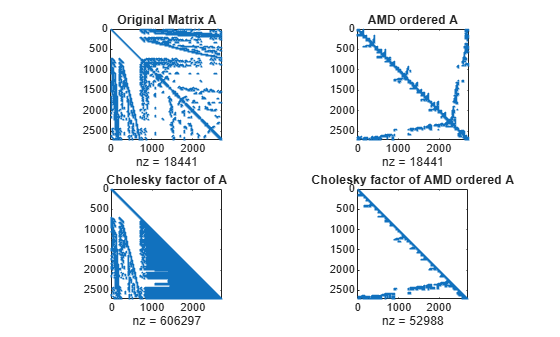# amd

Approximate minimum degree permutation

## Syntax

```P = amd(A) P = amd(A,opts) ```

## Description

`P = amd(A)` returns the approximate minimum degree permutation vector for the sparse matrix ```C = A + A'```. The Cholesky factorization of `C(P,P)` or `A(P,P)` tends to be sparser than that of `C` or `A`. The `amd` function tends to be faster than `symamd`, and also tends to return better orderings than `symamd`. Matrix `A` must be square. If `A` is a full matrix, then `amd(A)` is equivalent to `amd(sparse(A))`.

`P = amd(A,opts)` allows additional options for the reordering. The `opts` input is a structure with the two fields shown below. You only need to set the fields of interest:

• dense — A nonnegative scalar value that indicates what is considered to be dense. If A is n-by-n, then rows and columns with more than `max(16,(dense*sqrt(n)))` entries in `A + A'` are considered to be "dense" and are ignored during the ordering. MATLAB® software places these rows and columns last in the output permutation. The default value for this field is 10.0 if this option is not present.

• aggressive — A scalar value controlling aggressive absorption. If this field is set to a nonzero value, then aggressive absorption is performed. This is the default if this option is not present.

MATLAB software performs an assembly tree post-ordering, which is typically the same as an elimination tree post-ordering. It is not always identical because of the approximate degree update used, and because “dense” rows and columns do not take part in the post-order. It well-suited for a subsequent `chol` operation, however, If you require a precise elimination tree post-ordering, you can use the following code:

```P = amd(S); C = spones(S)+spones(S'); [ignore, Q] = etree(C(P,P)); P = P(Q);```

If `S` is already symmetric, omit the second line, `C = spones(S)+spones(S')`.

## Examples

collapse all

Compute the Cholesky factor of a matrix before and after it is ordered using `amd` to examine the effect on sparsity.

Load the barbell graph sparse matrix and add a sparse identity matrix to it to ensure it is positive definite. Compute two Cholesky factors: one of the original matrix and one of the original matrix preordered with `amd`.

```load barbellgraph.mat A = A+speye(size(A)); p = amd(A); L = chol(A,'lower'); Lp = chol(A(p,p),'lower');```

Plot the sparsity patterns of all four matrices. The Cholesky factor obtained from the preordered matrix is much sparser compared to the factor of the matrix in its natural ordering.

```figure subplot(2,2,1) spy(A) title('Original Matrix A') subplot(2,2,2) spy(A(p,p)) title('AMD ordered A') subplot(2,2,3) spy(L) title('Cholesky factor of A') subplot(2,2,4) spy(Lp) title('Cholesky factor of AMD ordered A')```